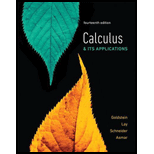## Solutions for Calculus & Its Applications (14th Edition)

### Book Details

Intuition before Formality. Calculus & Its Applications builds intuition with key concepts of calculus before the analytical material. For example, the authors explain the derivative geometrically before they present limits, and they introduce the definite integral intuitively via the notion of net change before they discuss Riemann sums. The strategic organization of topics makes it easy to adjust the level of theoretical material covered. The significant applications introduced early in the course serve to motivate students and make the mathematics more accessible. Another unique aspect of the text is its intuitive use of differential equations to model a variety of phenomena in Chapter 5, which addresses applications of exponential and logarithmic functions.

## More Editions of This Book

Corresponding editions of this textbook are also available below:

Student Solutions Manual for Calculus & Its Applications and Calculus & Its Applications, Brief Version
14th Edition
ISBN: 9780134463230
CALCULUS+ITS APPL..-MYLAB ACCESS
14th Edition
ISBN: 9780135901236
Calculus & Its Applications, 13/e
13th Edition
ISBN: 9780321848901
CALCULUS MYLAB MATH WITH EBOOK CODE
13th Edition
ISBN: 9780321878885